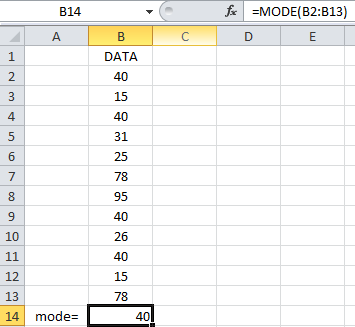## How To Calculate Mode In Excel

To learn how to calculate the mode in excel follow the steps below. We are going to use dummy data.Open Excel and create a new spreadsheet. Enter your dataPut the formula =mode (B2:B13) in the formula bar (fx).Press enter and that’s it.The mode has now being calculated.

## Template

```Further reading: